Case Based Questions (MCQ)

Chapter 2 Class 10 Polynomials
Serial order wise

## The below picture are few natural examples of parabolic shape which is represented by a quadratic polynomial. A parabolic arch is an arch in the shape of a parabola. In structures, their curve represents an efficient method of load, and so can be found in bridges and in architecture in a variety of forms.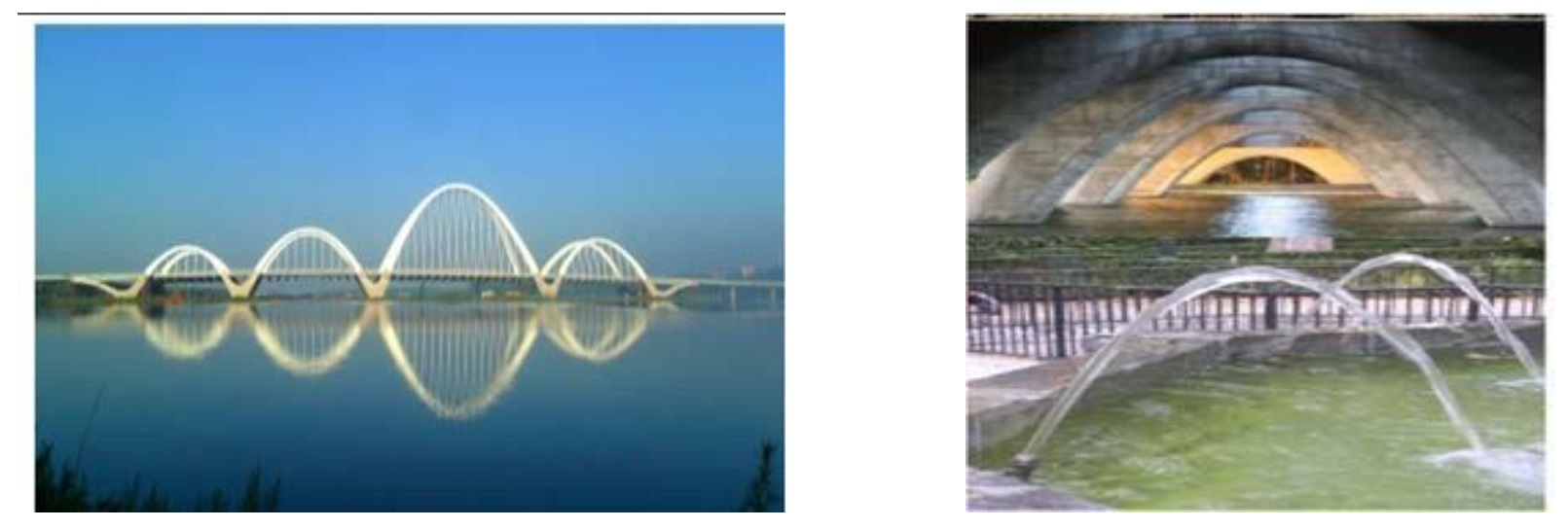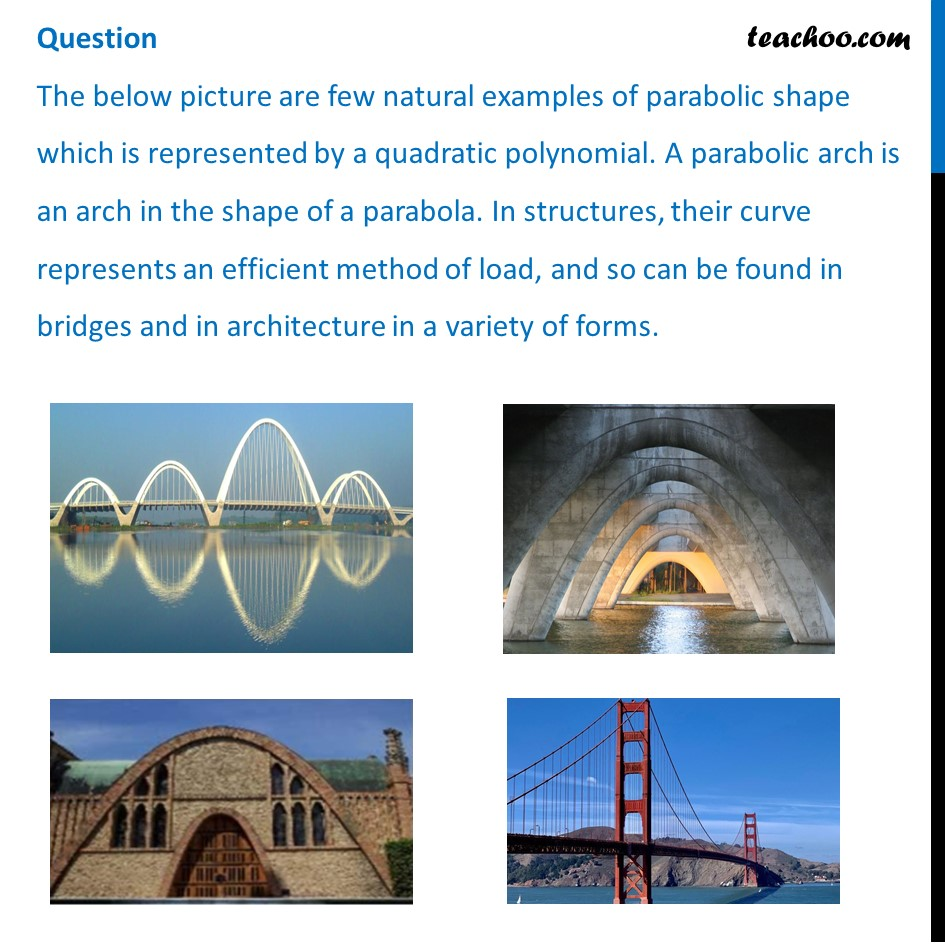## In the standard form of quadratic polynomial, 𝑎𝑥 2 +𝑏𝑥+𝑐   a, b and c are (a) All are real numbers   (b) All are rational numbers.  (c) ‘a’ is a non zero real number and b and c are any real numbers  (d) All are integers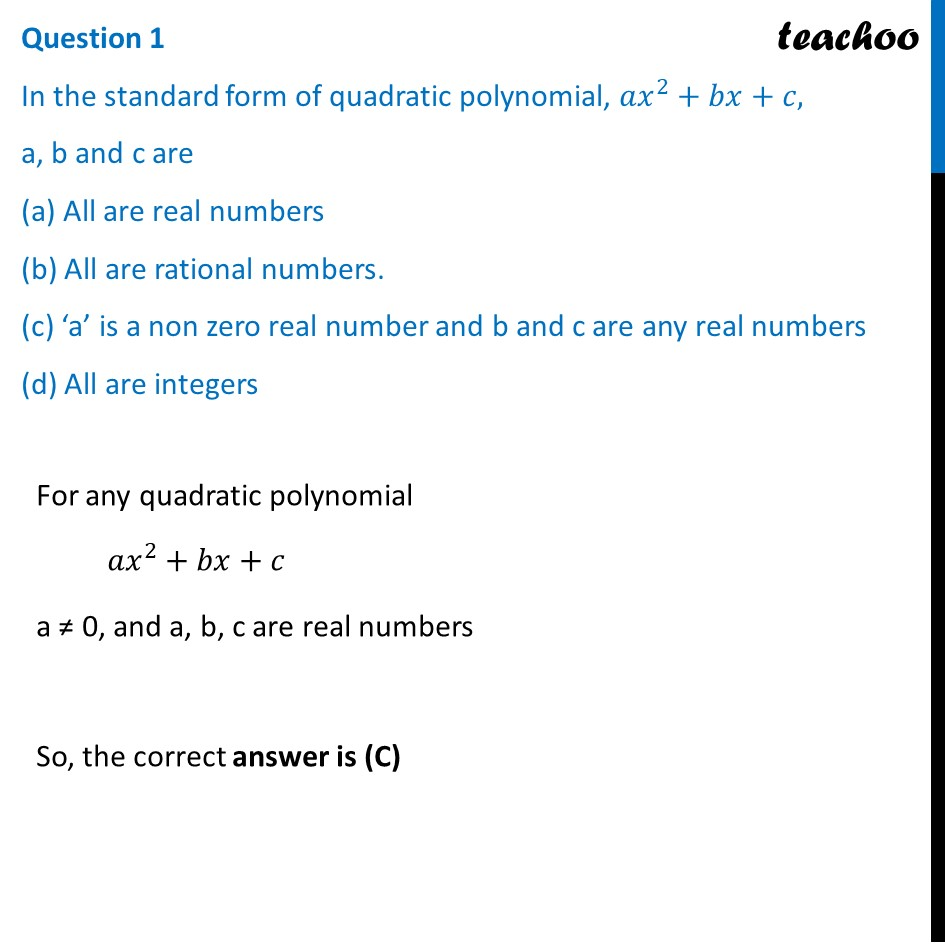## If the roots of the quadratic polynomial are equal, where the discriminant 𝐷=𝑏 2 −4𝑎𝑐, then (a) D > 0  (b) D < 0   (c) D ≥ 0   (d) D = 0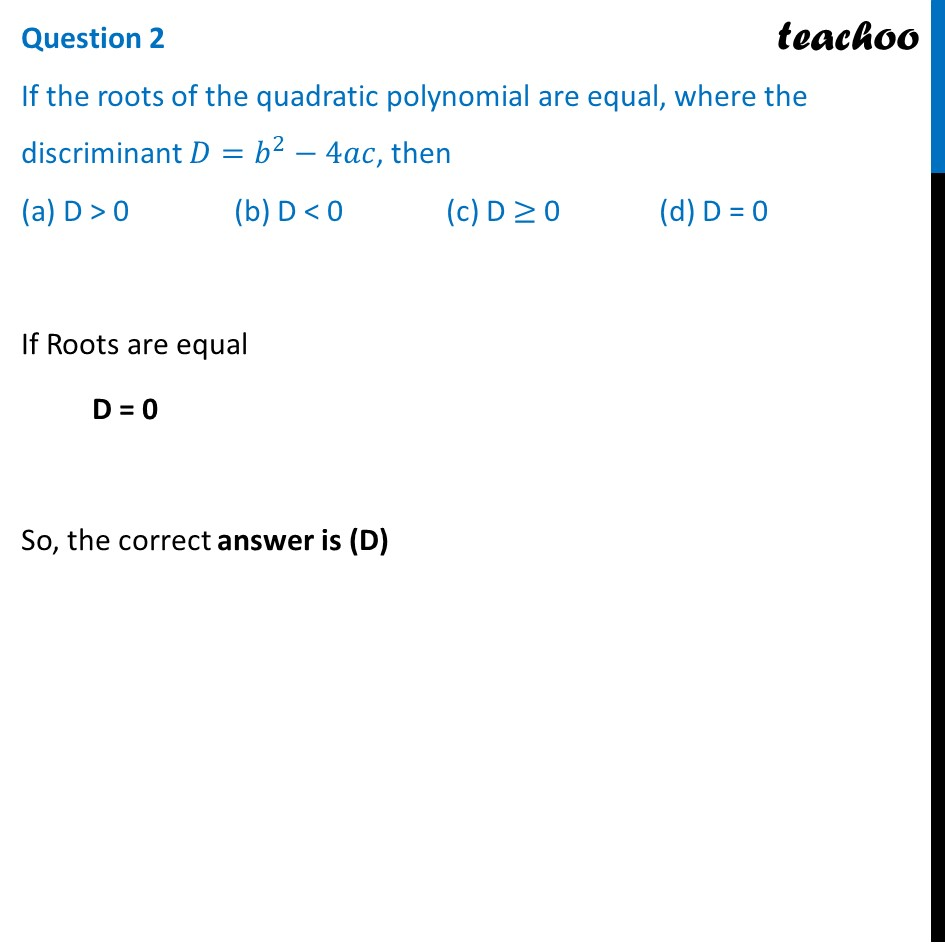## If a and 1/a are the zeroes of the quadratic polynomial 2𝑥 2 − 𝑥 + 8𝑘 then k is (a) 4  (b) 1/4  (c) (-1)/4    (d) 2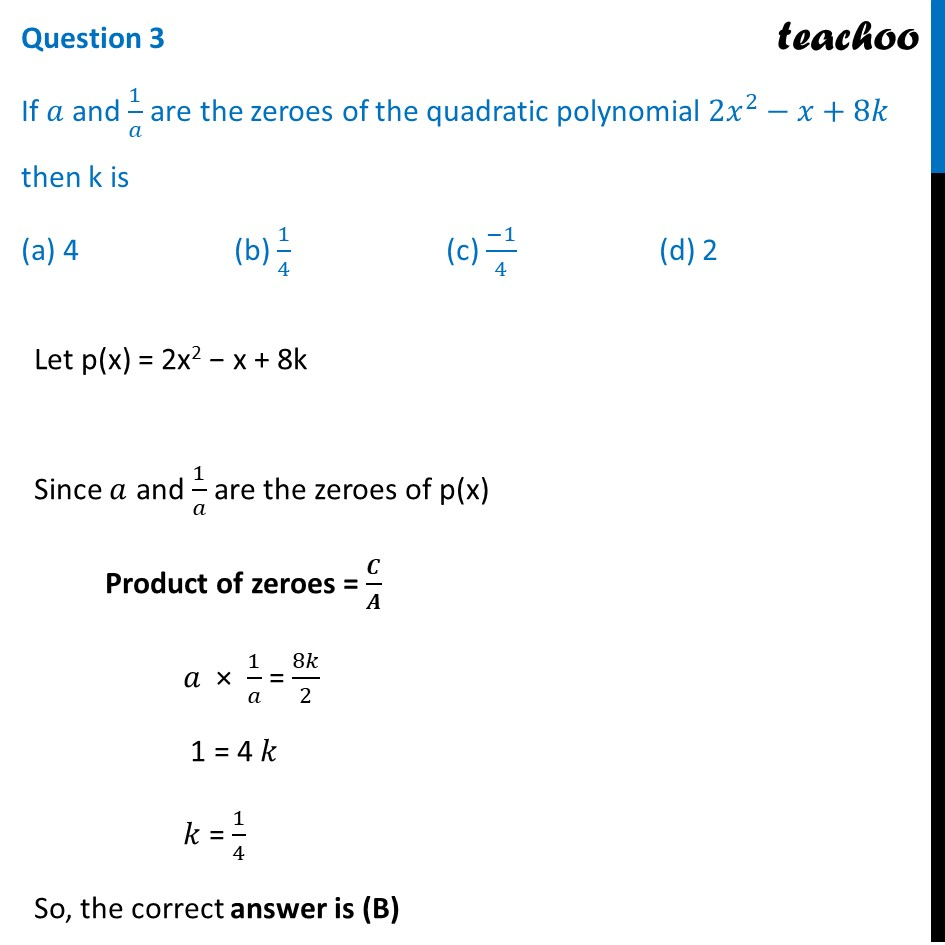## The graph of 𝑥 2 +1=0 (a) Intersects x‐axis at two distinct points.   (b) Touches x‐axis at a point.   (c) Neither touches nor intersects x‐axis.   (d) Either touches or intersects x‐ axis.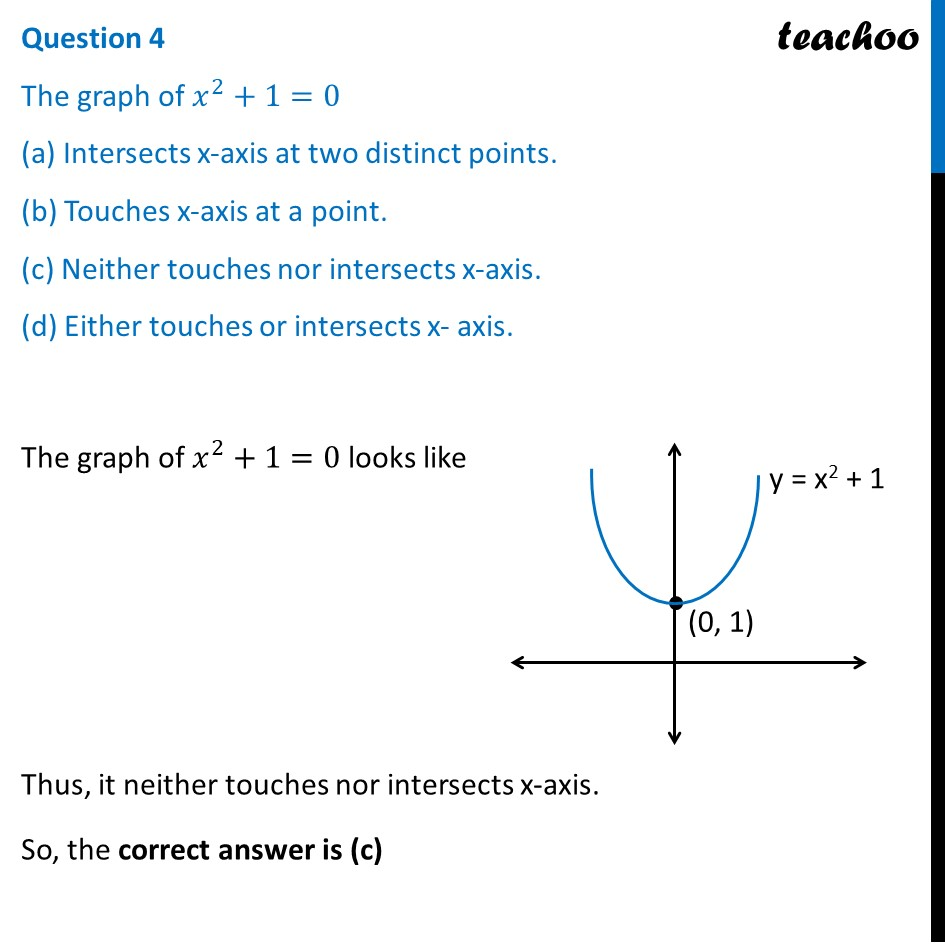## If the sum of the roots is –p and product of the roots is (-1)/p , then the quadratic polynomial is (a) 𝑘(−𝑝𝑥 2 +𝑥/𝑝+1)  (b) 𝑘(𝑝𝑥 2 −𝑥/𝑝−1)     (c) 𝑘(𝑥 2 +𝑝𝑥−1/𝑝)    (d) 𝑘(𝑥 2 −𝑝𝑥+1/𝑝)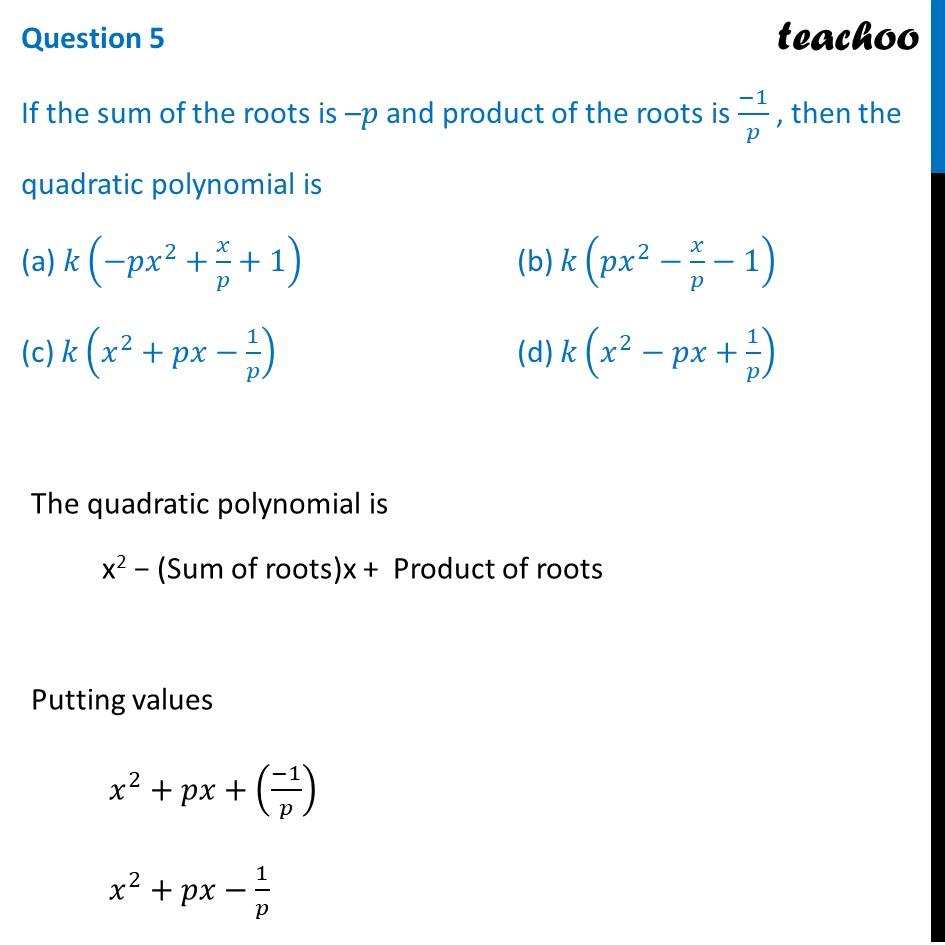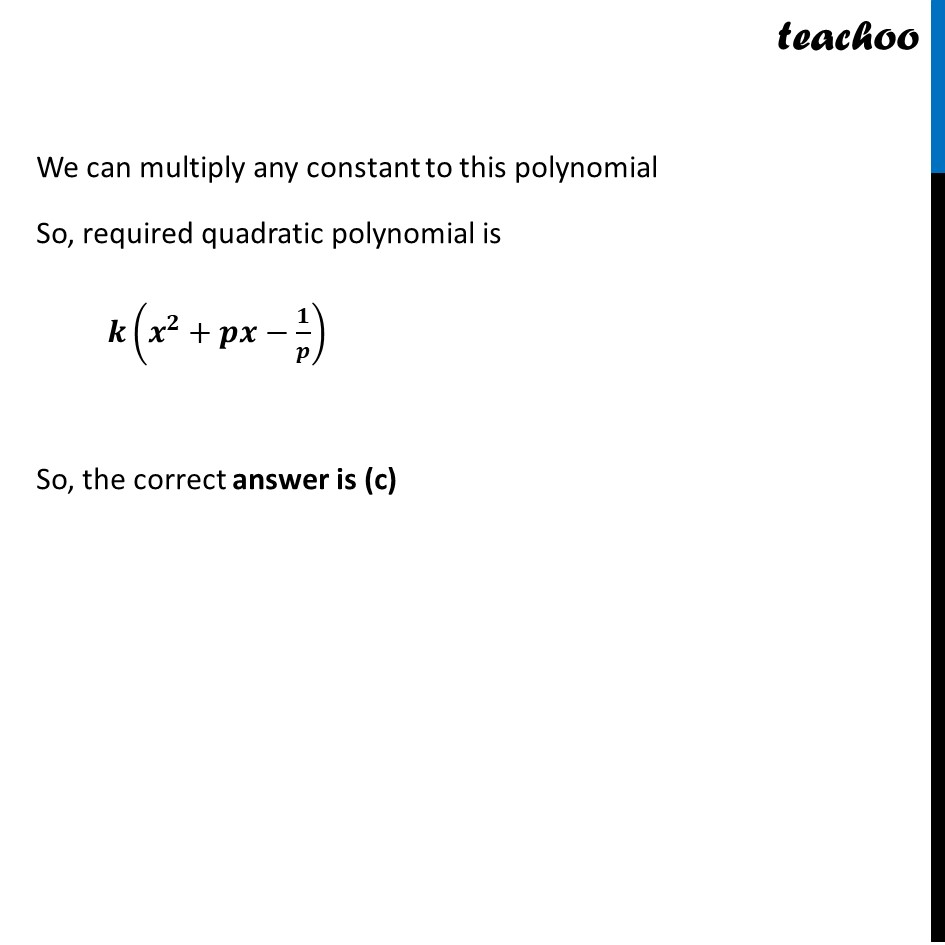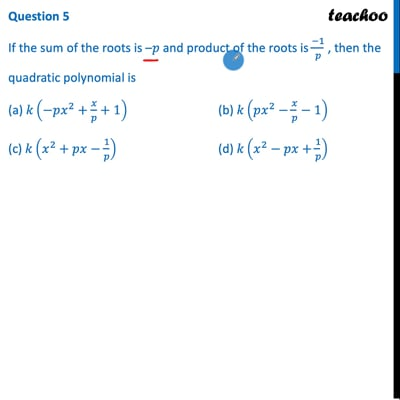This video is only available for Teachoo black users

Solve all your doubts with Teachoo Black (new monthly pack available now!)

### Transcript

Question The below picture are few natural examples of parabolic shape which is represented by a quadratic polynomial. A parabolic arch is an arch in the shape of a parabola. In structures, their curve represents an efficient method of load, and so can be found in bridges and in architecture in a variety of forms.Question 1 In the standard form of quadratic polynomial, 〖𝑎𝑥〗^2+𝑏𝑥+𝑐, a, b and c are (a) All are real numbers (b) All are rational numbers. (c) ‘a’ is a non zero real number and b and c are any real numbers (d) All are integers For any quadratic polynomial 〖𝑎𝑥〗^2+𝑏𝑥+𝑐 a ≠ 0, and a, b, c are real numbers So, the correct answer is (C) Question 2 If the roots of the quadratic polynomial are equal, where the discriminant 𝐷=𝑏^2−4𝑎𝑐, then (a) D > 0 (b) D < 0 (c) D ≥ 0 (d) D = 0 If Roots are equal D = 0 So, the correct answer is (D) Question 3 If 𝑎 and 1/𝑎 are the zeroes of the quadratic polynomial 2𝑥^2−𝑥+8𝑘 then k is (a) 4 (b) 1/4 (c) (−1)/4 (d) 2 Let p(x) = 2x2 − x + 8k Since 𝑎 and 1/𝑎 are the zeroes of p(x) Product of zeroes = 𝑪/𝑨 𝑎 × 1/𝑎 = 8𝑘/2 1 = 4 𝑘 𝑘 = 1/4 So, the correct answer is (B) Question 4 The graph of 𝑥^2+1=0 (a) Intersects x‐axis at two distinct points. (b) Touches x‐axis at a point. (c) Neither touches nor intersects x‐axis. (d) Either touches or intersects x‐ axis. The graph of 𝑥^2+1=0 looks like Thus, it neither touches nor intersects x‐axis. So, the correct answer is (c) Question 5 If the sum of the roots is –𝑝 and product of the roots is (−1)/𝑝 , then the quadratic polynomial is (a) 𝑘(−𝑝𝑥^2+𝑥/𝑝+1) (b) 𝑘(𝑝𝑥^2−𝑥/𝑝−1) (c) 𝑘(𝑥^2+𝑝𝑥−1/𝑝)" " (d) 𝑘(𝑥^2−𝑝𝑥+1/𝑝)" " The quadratic polynomial is x2 − (Sum of roots)x + Product of roots Putting values 𝑥^2+𝑝𝑥+((−1)/𝑝) 𝑥^2+𝑝𝑥−1/𝑝 We can multiply any constant to this polynomial So, required quadratic polynomial is 𝒌(𝒙^𝟐+𝒑𝒙−𝟏/𝒑) So, the correct answer is (c)